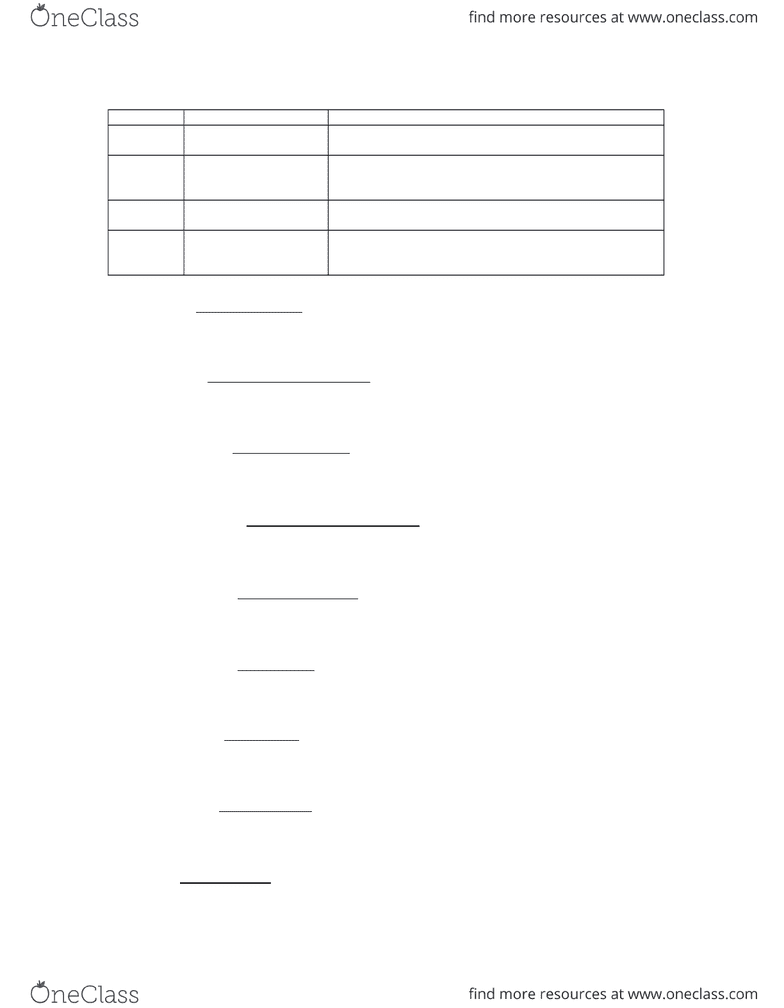Textbook Notes (270,000)
CA (160,000)
UTSG (10,000)
RSM100Y1 (400)
Chapter Appendix

# RSM100Y1 Chapter Notes - Chapter Appendix: Gross Margin, Asset, Inventory Turnover

Department
Rotman Commerce
Course Code
RSM100Y1
Professor
John Oesch
Chapter
Appendix

This preview shows half of the first page. to view the full 3 pages of the document.Major Categories of Financial Ratios
Category Ratio Description
Liquidity
ratios
Current ratio
Quick (acid-test) ratio
Current assets divided by current liabilities
Current assets (minus inventory) divided by current liabilities
Efficiency
ratios
Inventory turnover
Receivables turnover
Total asset turnover
Cost of goods sold divided by average inventory
Credit sales divided by average accounts receivable
Revenue or sales divided by average total assets
Leverage
ratios
Debt ratio
Long-term debt to equity
Total liabilities divided by total assets
Long-term debt divided by owners’ equity
Profitabilit
y ratios
Gross profit margin
Net profit margin
Return on equity
Gross profit divided by revenue or sales
Net profit divided by revenue or sales
Net profit divided by average owners’ equity
Current ratio =Current assets
Current liabilities
Acid-test ratio =Current assets - Inventory
Current liabilities
Inventory turnover =Cost of goods sold
Average inventory
Receivables turnover =Credit sales
Average accounts receivable
Total asset turnover =Sales
Average total assets
Gross profit margin =Gross profit
Sales
Net profit margin =Net income
Sales
Return on equity =Net income
Average equity
Debt ratio =Total liabilities
Total assets
###### You're Reading a Preview

Unlock to view full version